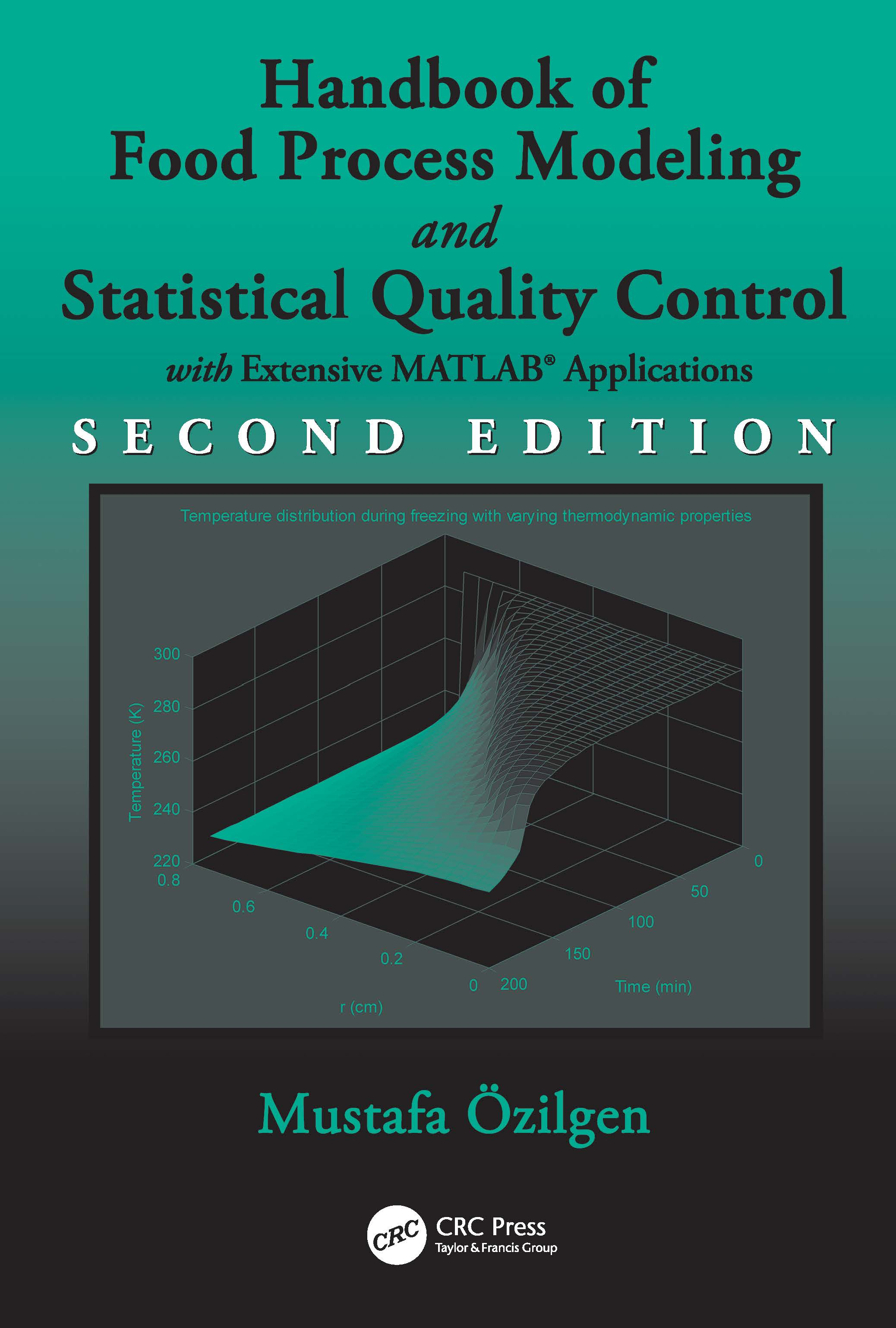# Handbook of Food Process Modeling and Statistical Quality Control

## 2nd Edition

CRC Press

704 pages | 276 B/W Illus.

##### Purchasing Options:\$ = USD
Hardback: 9781439814864
pub: 2011-03-01
SAVE ~\$50.00
\$250.00
\$200.00
x
eBook (VitalSource) : 9780429109607
pub: 2011-03-01
from \$28.98

FREE Standard Shipping!

### Description

Presenting mathematical prerequisites in summary tables, this book explains fundamental techniques of mathematical modeling processes essential to the food industry. The author focuses on providing an in-depth understanding of modeling techniques, rather than the finer mathematical points. Topics covered include modeling of transport phenomena, kinetic processes, and food engineering operations. The book also discusses statistical process analysis and quality control. It includes examples from almost every conceivable food process, most of which are based on real data given in the many references. Each example is followed by a clear, step-by-step worked solution.

### Table of Contents

Introduction to Process Modeling

The Property Balance

What is Process Modeling?

Empirical Models and Linear Regression

References.

Transport Phenomena Models

The Differential General Property Balance Equation

Equation of Continuity

Equation of Energy

Equation of Motion

Theories for Liquid Transport Coefficients

Analytical Solutions to Ordinary Differential Equations

Transport Phenomena Models Involving Partial Differential Equations

Chart Solutions to Unsteady State Conduction Problem.

Interfacial Mass Transfer

Rheological Modeling

The Engineering Bernoulli Equation

Laplace Transformations in Mathematical Modeling.

Numerical Methods in Mathematical Modeling

References

Kinetic Modeling.

Kinetics and Food Processing

The Rate Expression

Why Do the Chemicals React?

Temperature Effects on the Reaction Rates

Precision of Reaction Rate Constant and Activation Energy Determinations

Enzyme Catalyzed Reaction Kinetics.

Analogy Kinetic Models

Metabolic Process Engineering

Microbial Kinetics

Kinetics of Microbial Death

Ideal Reactor Design

References.

Mathematical Modeling in Food Engineering Operations

Thermal Process Modeling

Moving Boundary and Other Transport Phenomena Models for Processes Involving Phase Change

Kinetic Modeling of Crystallization Processes

Unit Operation Models

References

Statistical Process Analysis and Quality Control

Statistical Quality Control

Statistical Process Analysis

Quality Control Charts for Measurements

Quality Control Charts for Attributes

Acceptance Sampling by Attributes

Standard Sampling Plans for Attributes

HACCP and FMEA Principles

Quality Assurance and Improvement Through Mathematical Modeling

References

Index

### About the Originator

#### Mustafa Özilgen

Istanbul, , Turkey

Learn more about Mustafa Özilgen >>

### Subject Categories

##### BISAC Subject Codes/Headings:
HEA048000
HEALTH & FITNESS / Diet & Nutrition / General
TEC012000
TECHNOLOGY & ENGINEERING / Food Science